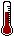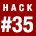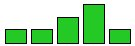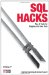# Hack 35. Tally Results into a ChartYou can show the results of a survey neatly using a bar chart that is much more informative than a simple average.

Suppose that users have been asked to rate web pages on a scale of one to five. The results have come in as follows:

```mysql> SELECT * FROM votes ORDER BY score;
+------------+-------+
| page | score |
+------------+-------+
| ms001.aspx | 1 |
| ms001.aspx | 2 |
| ms001.aspx | 3 |
| ms001.aspx | 3 |
| ms001.aspx | 4 |
| ms001.aspx | 4 |
| ms001.aspx | 4 |
| ms001.aspx | 5 |
+------------+-------+```

The mean score for this page is 3.25. To get the bar chart, first you need the total number of votes for each score. This query relies on a simple table called numbers that contains integers from 1 to 5 in a column called n:

```mysql> SELECT n, COUNT(score)
-> FROM numbers LEFT OUTER JOIN votes ON (n=score)
-> GROUP BY n;
+---+--------------+
| n | COUNT(score) |
+---+--------------+
| 1 | 1 |
| 2 | 1 |
| 3 | 2 |
| 4 | 3 |
| 5 | 1 |
+---+--------------+```

To represent this graphically you can use the REPEAT function:

```mysql> SELECT n, REPEAT('#',COUNT(score))
-> FROM numbers LEFT OUTER JOIN votes ON (n=score)
-> GROUP BY n;
+---+--------------------------+
| n | REPEAT('#',COUNT(score)) |
+---+--------------------------+
| 0 | |
| 1 | # |
| 2 | # |
| 3 | ## |
| 4 | ### |
| 5 | # |
+---+--------------------------+```

The REPEAT function is specific to MySQL, but each of the others has something that will do the same job, as shown in the following sections.

In SQL Server:

```SELECT n, REPLICATE('#',COUNT(score))
FROM numbers LEFT JOIN votes ON n=score
GROUP BY n;```

In Oracle:

```SELECT n, LPAD(' ',1+COUNT(score),'#')
FROM numbers LEFT JOIN votes ON n=score
GROUP BY n;```

In PostgreSQL:

```SELECT n, LPAD('',CAST(COUNT(score) AS INT),'#')
FROM numbers LEFT JOIN votes ON n=score
GROUP BY n;```

You can also normalize the values. If you multiply by 20 and then divide by the total number of votes cast, the largest bar can be no more than 20 units:

```mysql> SELECT n, REPEAT('#',COUNT(score)*20/tot)
-> FROM numbers LEFT JOIN votes ON n=score,
-> (SELECT COUNT(*) tot FROM votes) t
-> GROUP BY n, tot;
+---+---------------------------------+
| n | REPEAT('#',COUNT(score)*20/tot) |
+---+---------------------------------+
| 1 | ### |
| 2 | ### |
| 3 | ##### |
| 4 | ######## |
| 5 | ### |
+---+---------------------------------+```

You can do it in HTML if you prefer (see Figure 5-2). You can use a single-pixel GIF image and set the height and width of the image in SQL:

```mysql> SELECT
-> REPLACE('',
-> 'hh',hh) bars
-> FROM (SELECT n, COUNT(score)*100/tot hh
-> FROM numbers LEFT JOIN votes ON n=score,
-> (SELECT COUNT(*) tot FROM votes) t
-> WHERE n BETWEEN 1 AND 5
-> GROUP BY n
-> ) t
-> ;
+-----------------------------------------------------------------+
| bars |
+-----------------------------------------------------------------+
||
||
||
||
||
+-----------------------------------------------------------------+ ```

Figure 5-2. A simple bar chartSQL Hacks
ISBN: 0596527993
EAN: 2147483647
Year: 2004
Pages: 147